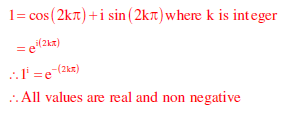# Engineering Mathematics - Online Test

Q1.
Consider the differential equation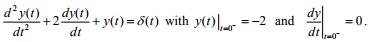The numerical value of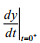is
Explaination / Solution: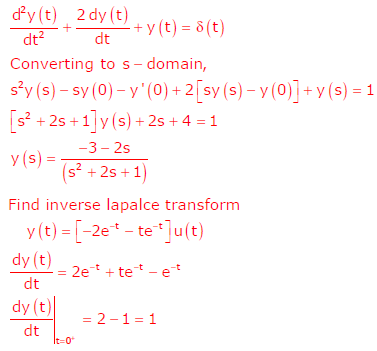Q2. A fair coin is tossed till a head appears for the first time. The probability that the number of required tosses is odd, is
Explaination / Solution: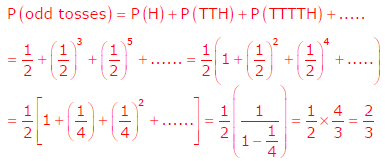Q3. The equation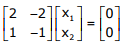has
Explaination / Solution:
No Explaination.

Q4. Square roots of − i, where i = √-1, are
Explaination / Solution:
No Explaination.

Q5. A function y = 5x2 + 10x is defined over an open interval x = (1,2). Atleast at one point in this interval, dy/dx is exactly
Explaination / Solution:
No Explaination.

Q6.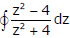evaluated anticlockwise around the circle |z - i| = 2, where i = √-1, is
Explaination / Solution:
No Explaination.

Q7. A matrix has eigenvalues –1 and –2. The corresponding eigenvectors are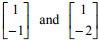respectively. The matrix is
Explaination / Solution:
No Explaination.

Q8. Which one of the following statements is true for all real symmetric matrices?
Explaination / Solution:

Eigen values of a real symmetric matrix are all real

Q9. Maximum of the real valued function f(x) = (x – 1)2/3 occurs at x equal to
Explaination / Solution: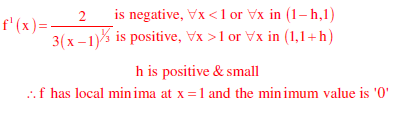Q10. All the values of the multi-valued complex function 1i, where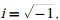Explaination / Solution: